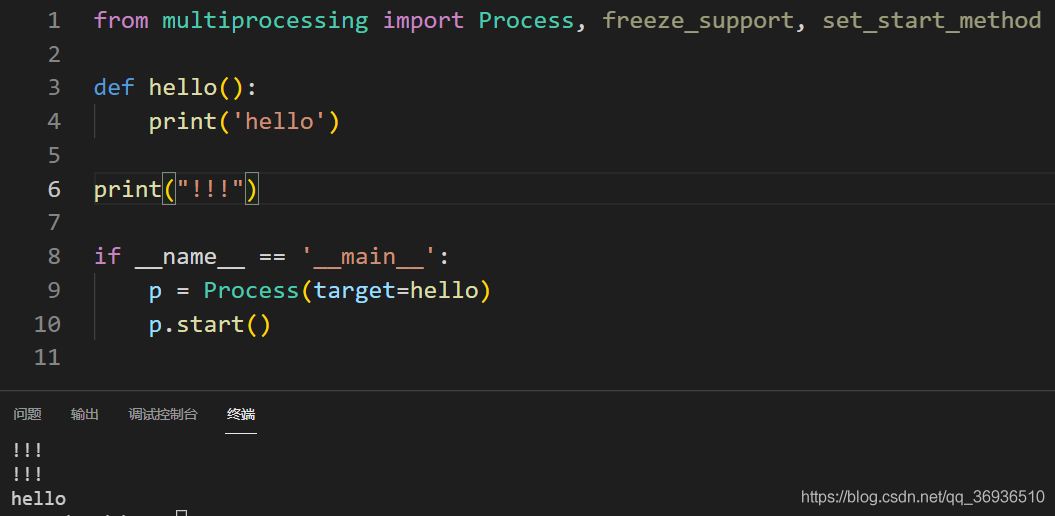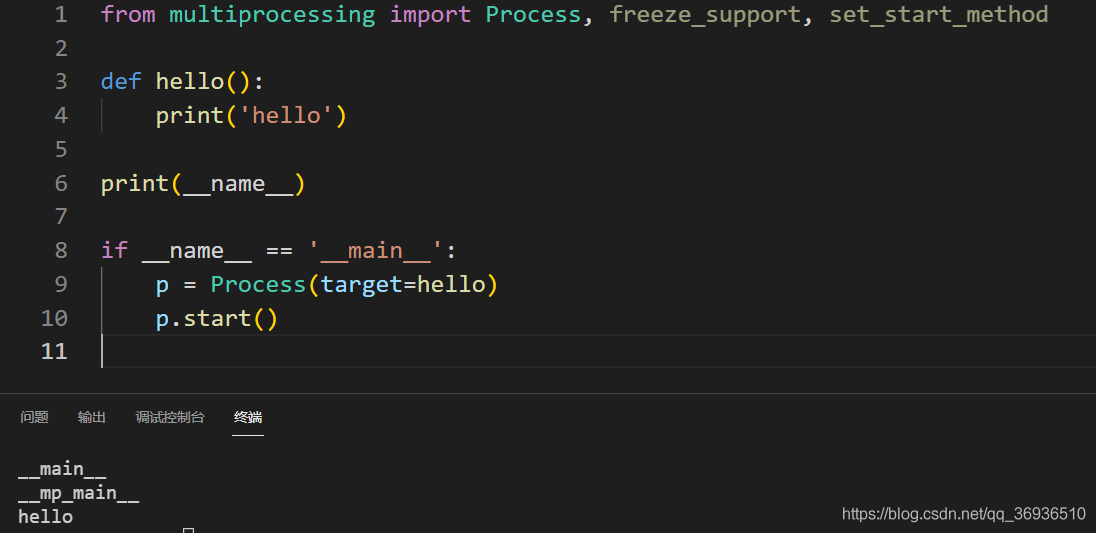# [Solved] RuntimeError (note: full exception trace is shown but execution is paused at: ＜module＞)

Error using multiprocessing module:

``````from multiprocessing import Process

def hello():
print('hello')

p = Process(target=hello)
p.start()
``````

Change the code to:

``````from multiprocessing import Process, freeze_support, set_start_method

def hello():
print('hello')

if __name__ == '__main__':
p = Process(target=hello)
p.start()
``````

Which `if __name__ == '__main__':`role is to protect the program entry point , when a sub-process using open Process, Python interpreter into the current module, and invoke the hello method, using a simple intuitive test:

``````from multiprocessing import Process, freeze_support, set_start_method

def hello():
print('hello')

print("!!!")

if __name__ == '__main__':
p = Process(target=hello)
p.start()
``````

In this code, ` Print ("!!"` is not a hello method. Let’s see the running result:`print("!!!")` was called twice!!! So before generating a new process, be sure to add ` if__ name__ == '__ main__':`

Further, if you change `print("!!!")`to `print(__name__)`, you will get the following results: